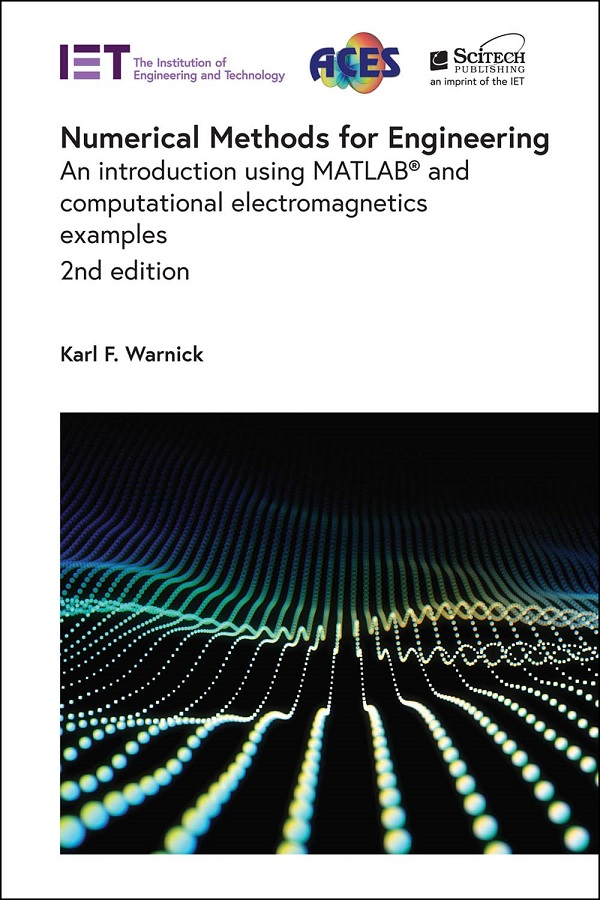In stock# Numerical Methods for Engineering, 2nd Edition

## An introduction using MATLAB® and computational electromagnetics examples

##### by Karl F. Warnick

The revised and updated second edition of this textbook teaches students to create modeling codes used to analyze, design, and optimize structures and systems used in wireless communications, microwave circuits, and other applications of electromagnetic fields and waves. Worked code examples are provided for key algorithms using the MATLAB technical computing language.

The book begins by introducing the field of numerical analysis and providing an overview of the fundamentals of electromagnetic field theory. Further chapters cover basic numerical tasks, finite difference methods, numerical integration, integral equations and the method of moments, solving linear systems, the finite element method, optimization methods, and inverse problems.

Developing and using numerical methods helps students to learn the theory of wave propagation in a concrete, visual, and hands-on way. This book fills the missing space of current textbooks that either lack depth on key topics or treat the topic at a level that is too advanced for undergraduates or first-year graduate students.

Presenting the topic with clear explanations, relevant examples, and problem sets that move from simple algorithms to complex codes with real-world capabilities, this book helps its readers develop the skills required for taking a mathematical prescription for a numerical method and translating it into a working, validated software code, providing a valuable resource for understanding the finite difference method, the method of moments, the finite element method, and other tools used in the RF and wireless industry.

Karl F. Warnick is a professor in the Department of Electrical and Computer Engineering at Brigham Young University, USA and a Fellow of the IEEE. He has published widely on electromagnetic theory, numerical methods, antenna applications, and high sensitivity phased arrays for satellite communications and radio astronomy.

Publication Year: 2020

Pages: 376

ISBN-13: 978-1-83953-073-9

Format: HBK